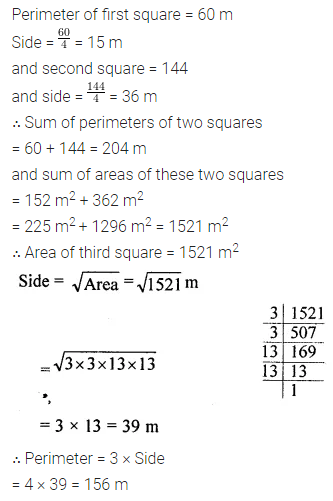## ML Aggarwal Class 8 Solutions for ICSE Maths Chapter 3 Squares and Square Roots Ex 3.3

Question 1.
By repeated subtraction of odd numbers starting from 1, find whether the following numbers are perfect squares or not? If the number is a perfect square then find its square root:
(i) 121
(ii) 55
(iii) 36
(iv) 90
Solution: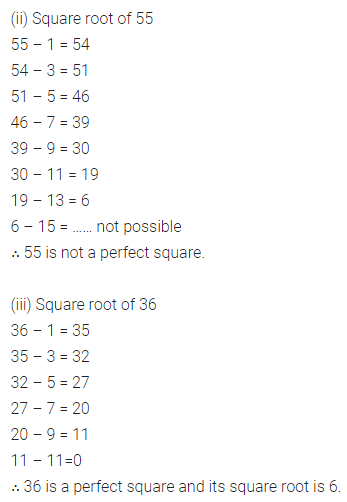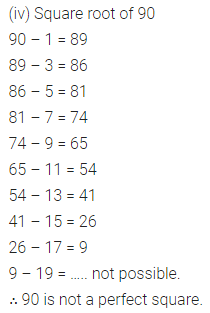Question 2.
Find the square roots of the following numbers by prime factorisation method:
(i) 784
(ii) 441
(iii) 1849
(iv) 4356
(v) 6241
(vi) 8836
(vii) 8281
(viii) 9025
Solution: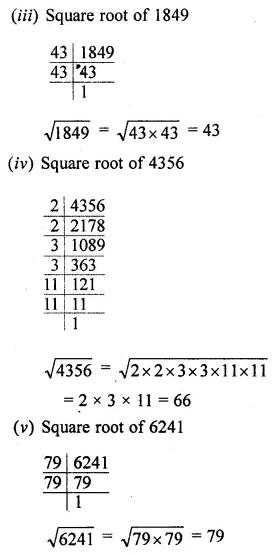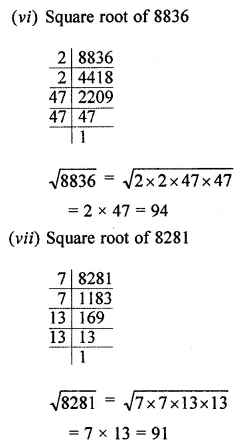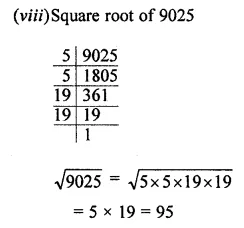Question 3.
Find the square roots of the following numbers by prime factorisation method: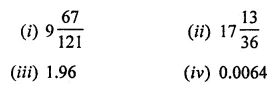Solution: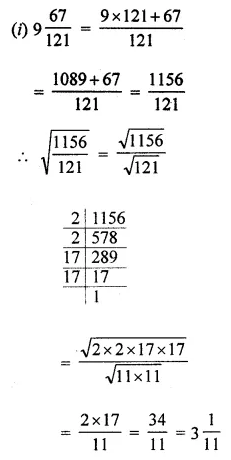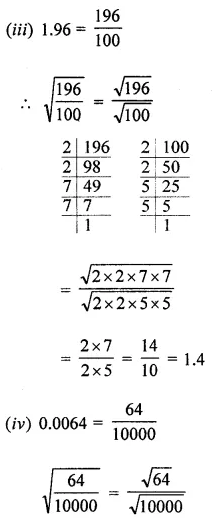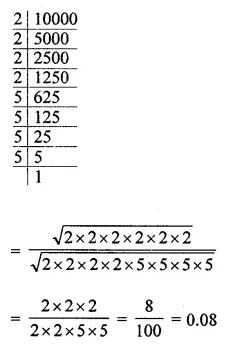Question 4.
For each of the following numbers, find the smallest natural number by which it should be multiplied so as to get a perfect square. Also, find the square root of the square number so obtained:
(i) 588
(ii) 720
(iii) 2178
(iv) 3042
(v) 6300
Solution: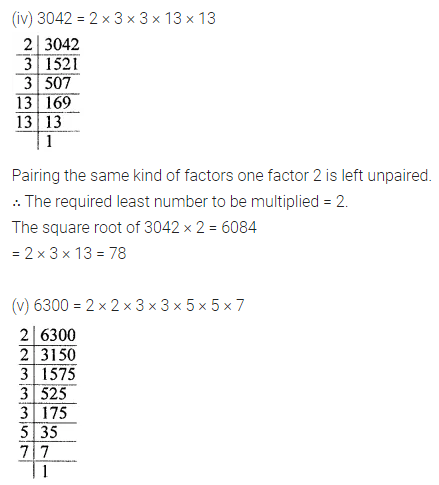Question 5.
For each of the following numbers, find the smallest natural number by which it should be divided so that this quotient is a perfect square. Also, find the square root of the square number so obtained:
(i) 1872
(ii) 2592
(iii) 3380
(iv) 16224
(v) 61347
Solution: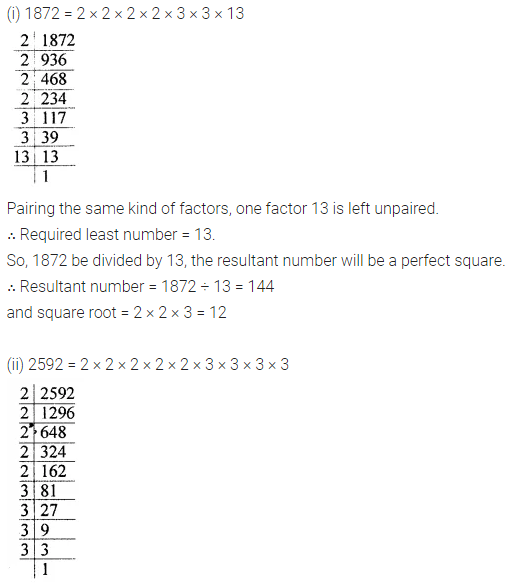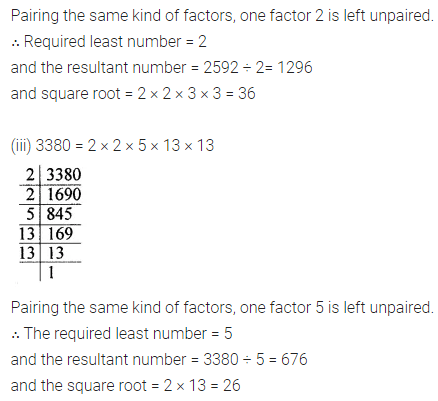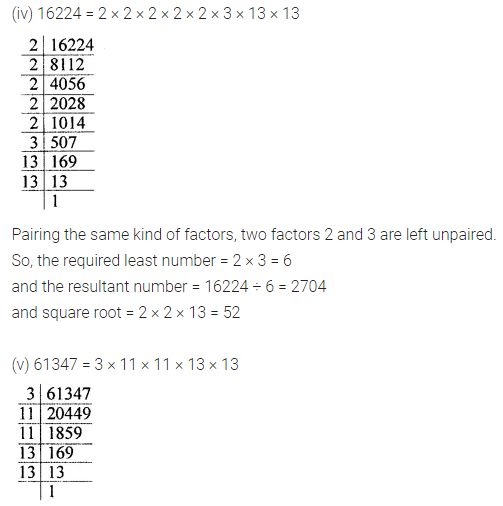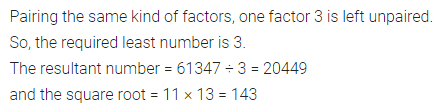Question 6.
Find the smallest square number that is divisible by each of the following numbers:
(i) 3, 6, 10, 15
(ii) 6, 9, 27, 36
(iii) 4, 7, 8, 16
Solution: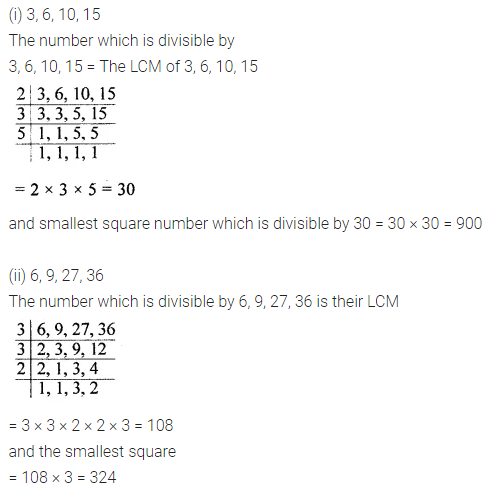Question 7.
4225 plants are to be planted in a garden in such a way that each row contains as many plants as the number of rows. Find the number of rows and the number of plants in each row.
Solution:Question 8.
The area of a rectangle is 1936 sq. m. If the length of the rectangle is 4 times its breadth, find the dimensions of the rectangle.
Solution: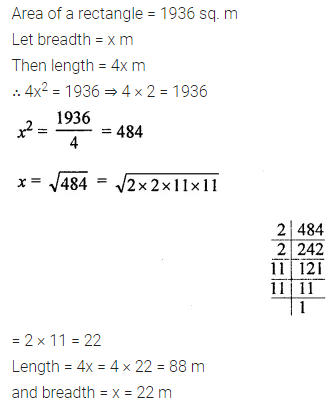Question 9.
In a school a P.T. teacher wants to arrange 2000 students in the form of rows and columns for P.T. display. If the number of rows is equal to number of columns and 64 students could not be accommodated in this arrangement. Find the number of rows.
Solution: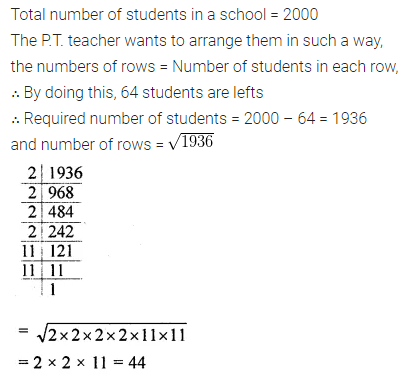Question 10.
In a school, the students of class VIII collected ₹2304 for a picnic. Each student contributed as mdny rupees as the number of students in the class. Find the number of students in the class.
Solution: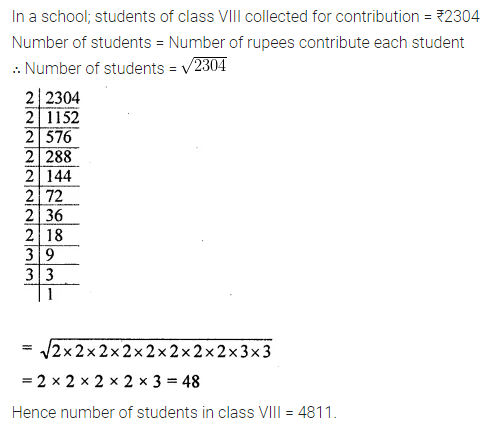Question 11.
The product of two numbers is 7260. If one number is 15 times the other number, find the numbers.
Solution: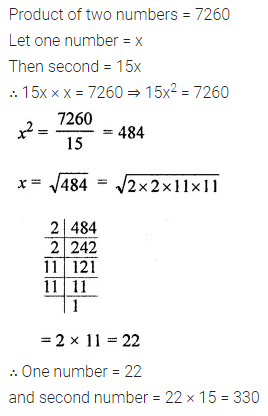Question 12.
Find three positive numbers in the ratio 2 : 3 : 5, the sum of whose squares is 950.
Solution: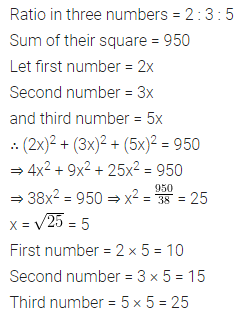Question 13.
The perimeter of two squares is 60 metres and 144 metres respectively. Find the perimeter of another square equal in area to the sum of the first two squares.
Solution: